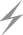Computer Aids for VLSI Design
Steven M. Rubin

Chapter 3: Representation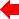Section 6 of 7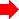## 3.6 Geometry Representation

The last aspect of VLSI design to be represented is its spatial dimensionality. There must be convenient ways to describe flat polygonal objects that are aggregated in parallel planes. In addition, the objects must be organized for efficient manipulation and search.

### 3.6.1 Shape, Transformation, and Graphics

Actual representation of geometry consists of shape, transformation, and graphical attributes. The shape attribute contains information about the actual drawing of the object and can be described in many ways (see Fig. 3.14). Given a shape-type attribute and an array of shape data parameters, any figure can be described. These shape parameters should be represented with integers because integer arithmetic is faster on many computers and does not suffer from uncertain precision. However, the programmer must beware of integer overflow, which goes unnoticed on most computers. Also, when using integers, the unit of spacing should be chosen to allow maximum accuracy, both now and in the future. For example, if a 1 × 1 square in the database represents 1 square micron, then submicron IC layout cannot be done with integers. The smallest representable unit should match the smallest unit size of the manufacturing device. Most IC mask-making equipment works at centimicron detail, so one unit should represent one-hundredth of a micron. In PC design, one unit should represent one micron. Be warned that very small units of representation will result in very large values, which can overflow even 32-bit integers, common on today's computers.

 Shape Type Shape Parameters Rectangle Center, size Polygon Point count, point coordinates Line Begin, end, thickness Circle Center, radius Arc Center, start, end Text Location, message, typeface
FIGURE 3.14 Shape description.

A common abstraction in design systems is to use a scalable layout unit that is independent of actual spacing. Mead and Conway coined the term lambda (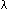) to describe a single unit of distance in the design. As the value ofvaries, the entire circuit scales. Spacing is usually expressed in integer multiples of, although half and quarterunits may be used. Besides agrid, it is also convenient to have coarser spacings for certain tasks. Several researchers have independently found that an 8grid is appropriate for the placement of cells [Schediwy; Sproull and Sutherland].

One issue that arises in the description of polygon shape is how to handle complex polygons such as those that intersect themselves. Although there are many solutions to this problem, one stands out as the most reasonable, especially for VLSI design [Newell and Sequin]. A point is defined to be inside a polygon if it has a positive (nonzero) winding number. The winding number is the number of times that another point, traveling around the perimeter of the polygon, wraps around the point in question. Besides this formal definition, Newell and Sequin show how complex polygons can be drawn and decomposed into simpler polygons.

Transformation attributes indicate modifications to the shape data. Transformations are typically linear, which means that the shape can be rotated, translated, or scaled. In a two-dimensional domain, this information can be represented with a 3 × 3 matrix of floating-point values. Since homogeneous coordinate transformations are not used in circuit design, only the left six elements of this matrix are valid [Newman and Sproull]. However, square matrices are easier to manipulate, so they continue to be used.

Another transformation that is rarely used in VLSI design is scaling. This is because object scaling is mostly done by modifying the shape coordinates rather than the transformations, and screen scaling is separate from the object representation. If scaling is eliminated, then the array elements will all fall into the range of zero to one and can be stored as fixed-point integers. This makes transformation operations faster on most computers. Thus a transformation matrix containing translation and rotation can be represented with six integers. Chapter 9, Graphics, discusses these transformations more fully.

The final aspect of geometric representation is graphical attributes that describe the visual qualities of an object. Examples are shown in Fig. 3.15. Note that this is different from shape because these graphical attributes are independent of coordinate values and can apply to any geometric object. Graphics attributes describe an object so that it can be distinguished from others that it overlaps. With the three geometric attributes of shape, transformation, and graphics any geometric object can be described.

 Attribute Parameters Color Red, green, blue Texture Pattern Filled-in Boolean Mask layer Layer number
FIGURE 3.15 Graphical attributes.

### 3.6.2 Orientation Restriction

One limitation that is often found in VLSI design is a restriction on the possible orientations that an object may have. Since arbitrary rotation requires transcendental mathematics, systems that allow them are usually more complex than those that allow only a fixed set of orientations. A limit to the possible orientations will make the representation more compact and faster. However a limit should be used only if the design environment lends itself to such restrictions.

In some VLSI layout, all geometry must be Manhattan, which means that the edges are parallel to the x and y axes. Every polygon is a rectangle and can be oriented in only one of eight ways (see Fig. 3.16). If the rectangle is uniform in its contents, no transformation is necessary since all orientations are merely variations on the shape. It is only when transforming a detailed component or a cell instance that these eight orientations are used.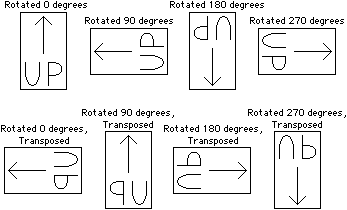FIGURE 3.16 Manhattan orientations.

In other VLSI design environments it is necessary to provide 45-degree angles. This is double the number of orientations in Manhattan geometry and is essentially the limit at which such layout is done. Although some synthesis tools generate geometry at arbitrary angles, sometimes called Boston geometry [Bryant], designers rarely use such a facility. The reason is that arbitrary-angle design rules do not exist for many IC processes and, if they did, would be so difficult to check that only a computer could produce error-free layout.

In non-IC environments such as schematic design, the geometry can appear at arbitrary angles. However, even Manhattan rules can be used and are often preferred because they make the layout look cleaner (see Fig. 3.17). If total flexibility of orientation is desired, the Manhattan rotations can still be coded as special cases. Full transformations can then be represented with 3 × 3 matrices, but one of the unused entries in the matrix indicates the special cases of Manhattan orientation.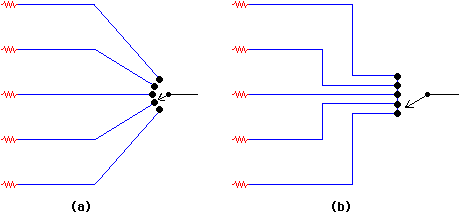FIGURE 3.17 Geometry restrictions dictate the appearance of the layout: (a) Arbitrary angles (b) Manhattan.

### 3.6.3 Geometry Algebra

To go beyond the basic attributes of geometric representation, it is necessary to consider the kinds of operations that will be performed on these objects. One such operation is geometry algebra. This set of operation performs union, intersection, expansion, and contraction on object shapes. Union operations are typically used to merge two adjoining shapes into one. Intersection is used to find the common area of two shapes, often for the purpose of eliminating redundancy. Expansion and contraction operations push the edges of a shape away from or toward the center.

Combinations of these operations can be very powerful. For example, design-rule checking is often done by expanding an object by its minimum spacing distance and then looking for areas of intersection with other objects. These areas are the design-rule violations. Expansion and contraction are used in compensation: a preprocessing step to fabrication that accounts for inaccuracies in manufacturing. There are even VLSI databases that require these algebra functions. For example, Caesar [Ousterhout 81] represents rectangles in its database and uses intersection tests to keep the rectangles nonredundant whenever changes are made. Nonredundant databases are occasionally required and often preferred. This is because they store less information and their analysis takes less time.

There are many forms of shape representation and each has its own methods of being algebraically manipulated. Manhattan rectangles are simple to modify. Polygons with 45-degree sides are more complex [Lanfri]. Arbitrary-angle polygons are the most complex but are often approximated with stair-stepped Manhattan rectangles [Batali and Hartheimer]. Circles, arcs, and conics demand a separate set of geometric algorithms, and become even more complex when mixed with polygonal representations. Here again, many curved shapes are approximated polygonally [Whitney]. The user should be allowed to have control over the grain of curve approximation so that the necessary smoothness can be produced.

The Polygon Package [Barton and Buchanan] performs all the geometric algebra functions and, in addition, allows both straight and curved lines to be used in the description of polygon boundaries. Sheets, which are multiple collections of these lines, can be aggregated to define polygons with holes. The most interesting representational aspect of this system is the organization of pairs of adjacent lines, sets of two pairs, sets of four pairs, and so on into a binary tree. The top of each tree encloses an entire polygon and each lower level of the tree has a bounding box that encloses its lines. This allows a search to rapidly find the specific lines involved in polygon intersections.

Another useful technique for storing polygonal information is winged-edge representation [Baumgart]. This representation captures the interrelation of polygons by sharing common edges and vertices. Thus a line on the border of two polygons will point to each one and will also be linked to the previous and subsequent edges on both polygons. This abundance of information is particularly useful in three-dimensional polygonal representations and can be simplified somewhat in the two-dimensional CAD world.

### 3.6.4 Search

Search is the operation that places the greatest demands on the geometric representation. Two operations that are important are the search for the neighbors of an object and the search for all objects at a given point or area. The way to make this efficient is to store sorted adjacency in the representation so that this information does not have to be determined during the search. Such adjacency will take time to compute when changes are made but will save much time later.

The problem is to determine what form of adjacency to represent. If the geometric objects are sorted by their center, then there is no information about their extent, and the relational information will not properly convey object location. For example, Fig. 3.18 shows two polygons the center coordinates of which do not indicate a spatial ordering in the x axis. The correct information to represent is the boundaries. By storing sorted edges, a walk through the edge list will precisely identify the objects in a given neighborhood.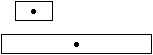FIGURE 3.18 Center-based object ordering is useless for a search.

The simplest representation of sorted edges is a linked list of objects according to edge order. One list can order the edges along only one axis. Therefore two lists are needed: one to cover horizontal edges and a second list to cover vertical edges. In some circumstances, the lists may need to distinguish between the two sides of an object. This means that the horizontal-edge list is actually two lists: one with the top edges and one with the bottom edges. For nonrectangular objects, a minimum bounding rectangle is used. These lists can be used to delimit a neighborhood because, when the near edge of an object extends beyond the bounds of the search, then the remaining objects on that direction's list are also out of range. For example, when searching for all objects in the range of 5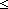x10, the program searches the left list until all objects bounds are less than 5 and then searches the right list until all objects bounds are greater than 10. The problem with this technique is that every object is represented multiple times and so it will be examined repeatedly during the search. Thus when a search for all objects in an area is conducted, the left and right lists will give no information about the vertical position of an object, so a scan of these lists will not limit itself in the up and down directions. Only by clever use of these lists can waste be prevented. For example, if the area of search is taller than it is wide, then the left- and right-edge lists should be used since they will do a better job of selection.

Another representation for spatial organization is called corner stitching [Ousterhout 84]. In it, all space in a layout is stored as a perfectly tiled area of nonoverlapping rectangles. Even the empty spaces have rectangles delimiting them. These area rectangles then have pointers that connect their corners to the adjacent area rectangles: The lower-left corner points to the rectangles below and to the left; the upper-right corner points to the rectangles above and to the right (see Fig. 3.19). With this representation, it is fairly simple to find the neighborhood of an object and to walk through the pointers in search of an object at a selected coordinate. However, it is necessary to use multiple "planes" of these rectangles in order to represent layout that overlaps on different layers. Also, the representation is mostly intended for Manhattan geometry and becomes complex when used otherwise.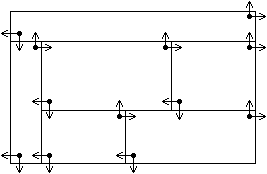FIGURE 3.19 Corner stitching.

Perhaps the best representation for the storage and search of spatial objects is the use of R-trees [Guttman]. This structure is a height-balanced tree in which all leaf nodes are at the same depth and contain spatial objects. Nodes higher in the tree also store boundary information that tightly encloses the leaf objects below. All nodes hold from M to 2M entries, where M is typically 25. The bounding boxes of two nodes may overlap, which allows arbitrary structures to be stored (see Fig. 3.20). A search for a point or an area is a simple recursive walk through the tree to collect appropriate leaf nodes.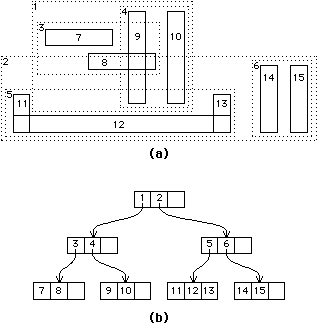FIGURE 3.20 R-tree organization: (a) Spatial ordering (b) Tree representation.

Insertion and deletion can be complex operations for R-trees. To insert a new object, the tree is searched from the top; at each level, the node that would expand least by the addition is chosen. When the bottom is reached, the object is added to the list if it fits. When there is no more room, the bottom node must be split in two, which adds a new element to the next higher node. This may recurse to the top, in which case the tree becomes deeper. Splitting a node is done by finding the two objects farthest apart along any dimension, and then clustering the remaining objects according to the rule of minimal boundary expansion.

R-tree deletion is done simply by removing the entry. If, however, the removal causes the node to have too few objects (less than M), then the entire node is deleted and the remaining valid objects are reinserted with the algorithm described in the previous paragraph. Deletion of a node may cause the next higher node also to contain too few objects, in which case the deletion recurses and may result in a shortened tree. Object-size updates require deletion and reinsertion.

Regardless of the spatial representation, search can be aided by providing an initial guess as to which objects are in the search area. When the search involves finding the neighbors of a particular object, that object is useful as the initial guess. When the object at a specified coordinate is sought, the most recently selected object can be used as the initial guess for the search; designers often work within a small area, so it is safe to assume that the next object selected will be close to the last object selected. This is the principle of locality and it applies to many tasks.

Speedy search requires that large amounts of information be available and rapidly accessible. This information must be organized well and the initial conditions for search must be favorable in order to achieve that speed. Efficient search is needed by many aspects of design and is crucial to a good design system.Previous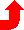Table of Contents NextSteven M. Rubin Static Free Software